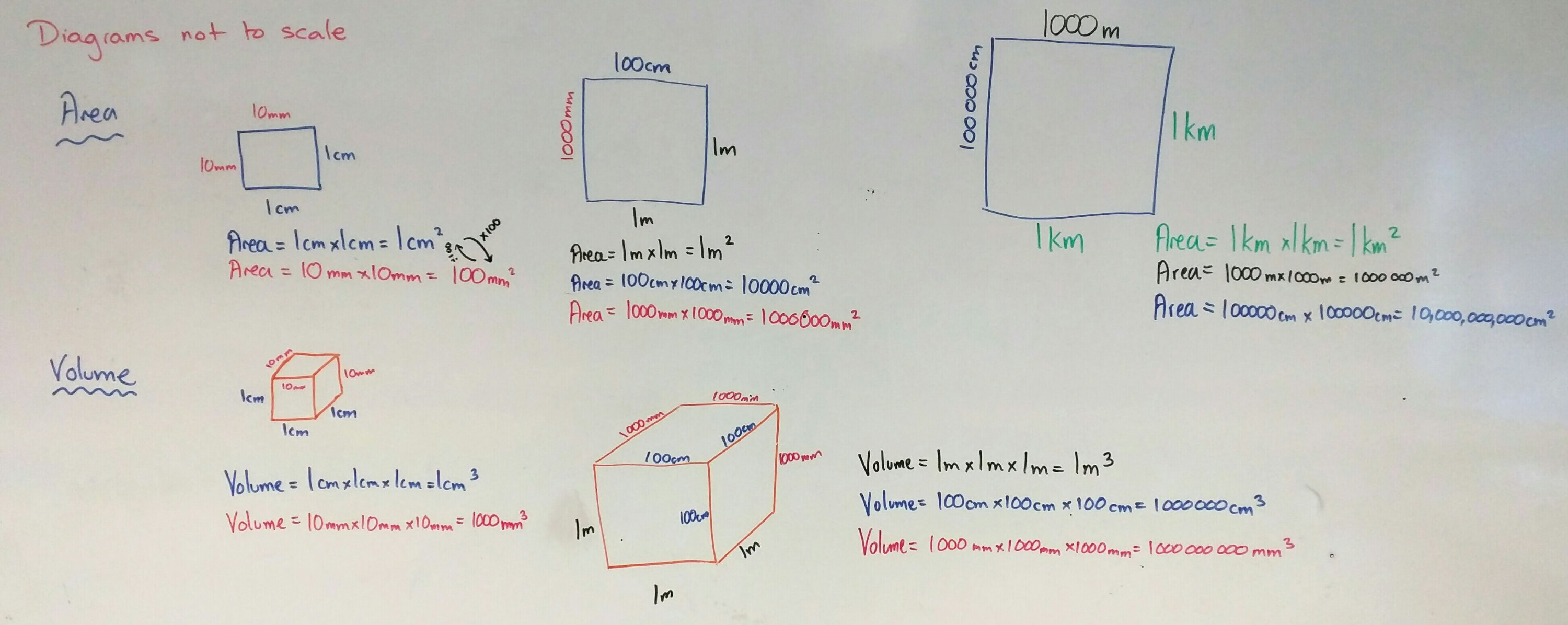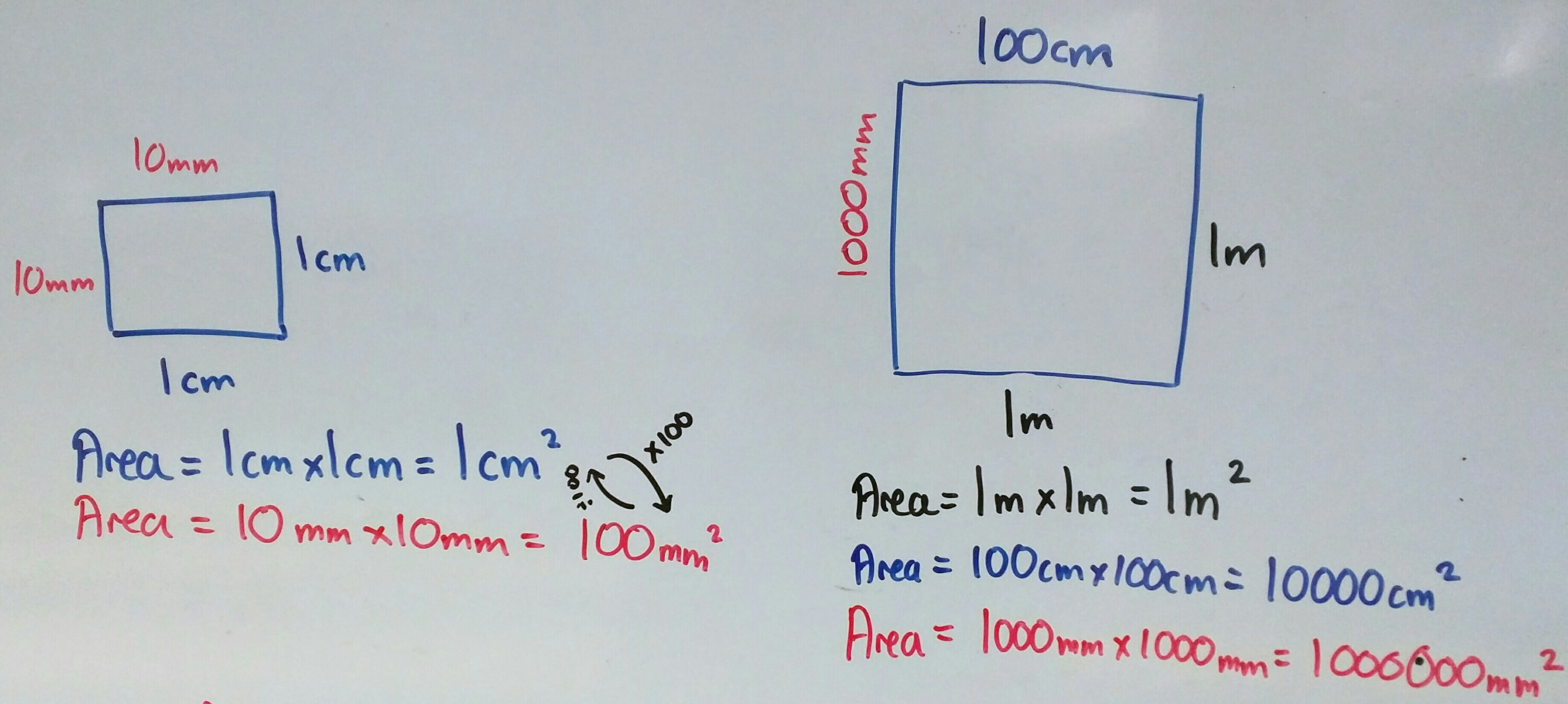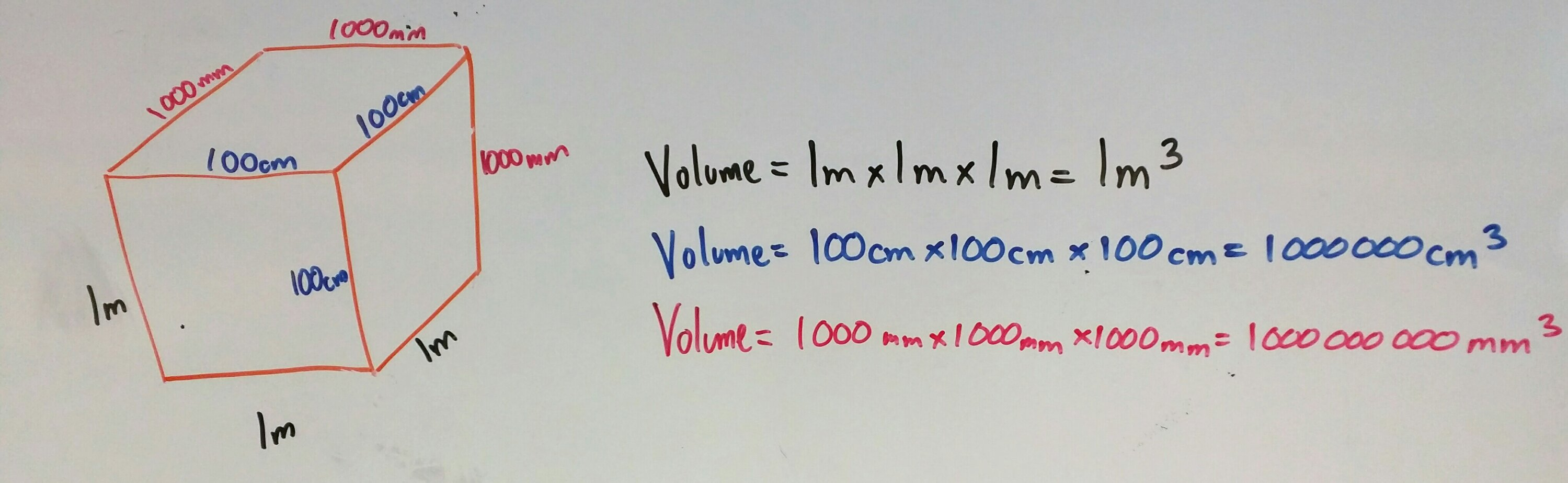# 361. Routes, Reindeer & Reasoning

Well, we are nearly at the end of a very crazy year. Congratulations on surviving it!

So, it’s been a while since the last blog post. Apologies for that. At the moment I am involved in Mixed Attainment teaching with Year 8. To finish off the term, I thought we deserved a bit of fun. We have a week of lessons left so I’m going for a mini project each lesson.

Lesson 1: Santa’s Route
I found this fab task on the Maths Drill website. There is a real chance for extension in this task, which is great for the mixed attainment classroom.

Lesson 2: Reindeer Ratios (Updated 13th Dec)
We have been following the White Rose Maths scheme for Year 8, which covers a lot of proportion and reasoning through ratio, multiplicative change and fractions. This task tries to cover some of these skills. The answers will be uploaded soon.

Lesson 3: Elf Box Packing Problem (Updated 14th Dec) Elf Box Packing Problem Solutions
This task involves using multiplicative change and fractional multiplication and division, with a dash of unit conversion. There is some work on shapes, but formulae are given where necessary. The first four pages print nicely into a folded A4 (A5) booklet. There is a help sheet for the box packing problem; this would be better printed on A4.

# 346. Area & Volume conversion

This is a quick post on how I teach metric unit conversion for area and volume. All you need is a big whiteboard and coloured board pens.Start by stressing that all diagrams are not to scale/accurate.

Two colours

1. Draw a square on the board
2. Pen colour 1: Label it as 1cm
3. What is the area? Show the calculation
4. What is 1cm in mm?
5. Pen colour 2: Label it as 10mm
6. What is the area in mm? Show the calculation
7. What is the scale factor between the sides? the area? why?Three colours

1. Draw a square on the board
2. Pen colour 3: Label it as 1m
3. What is the area? Show the calculation
4. What is 1m in cm?
5. Pen colour 2: Label it as 100cm
6. What is the area in cm? Show the calculation
7. What is the scale factor between the sides? the area? why?
8. Repeat in pen colour 1 for mm

Four colours

Well not actually four colours – pens 2,3 & 4 only. Repeat the process for kilometres to metres and centimetres.

Volume – same process, just three dimensionsWhy all the colours?

By coding each unit of measurement with a colour students can see the progression of the calculations and the links between area/volume and scale factor. After all, an okay mathematician can reproduce memorised facts, but a great mathematician doesn’t need to memorise – they understand where the calculations came from.

# 26. FDP Pyramid

This nifty little pyramid summarises how to convert between fractions, decimals and percentages.

Equipment
A5 paper or lightweight card
Scissors
Pens
Glue/tape
Compasses & pencil

Make a square
Fold the paper over to make a perfect 45 degree angle. Cut off the excess paper to make a square.

Fold & Cut
Unfold the square and fold the opposite diagonal. Cut from one corner to the middle along the fold.

Label
Draw an X on one of the quarters next to the cut. You will glue this piece later.

Either side of each fold label ‘Fraction’, ‘Percent’, ‘Decimal’.

Arrows
Using compasses and pencil, lightly draw two circles. Go over these lines with a pen to create one set of arrows going clockwise and one set anti-clockwise.

Facts
Label each arrow with the correct conversion fact and example.

Stick
Fold the X flap behind the next section and glue in place.

Summary
This is a tactile activity which could be used on a wall display. It can also be collapsed down flat where it can be taped on one side into a book and ‘pop up’ when required.